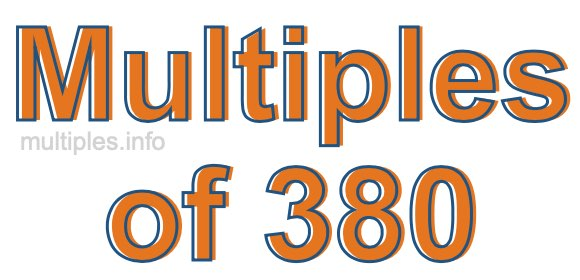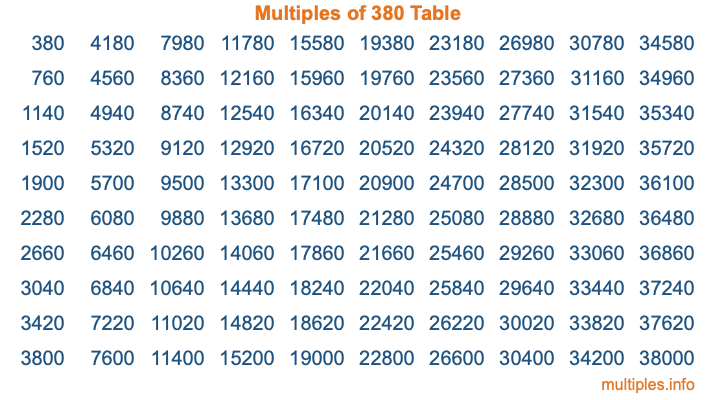Multiples of 380Welcome to the Multiples of 380 page. Here we will first teach you everything you will ever need to know about the multiples of 380, and then give you a study guide summary of everything we taught you to make sure you remember it all. Use this page to look up facts and learn information about the multiples of 380. This page will make you a multiples of three hundred eighty expert!

Definition of Multiples of 380
Multiples of 380 are all the numbers that when divided by 380 equal an integer. Each of the multiples of 380 are called a multiple. A multiple of 380 is created by multiplying 380 by an integer.

Therefore, to create a list of multiples of 380, you start with 1 multiplied by 380, then 2 multiplied by 380, then 3 multiplied by 380, and so on for as long as you want. Thus, the list of the first five multiples of 380 is 380, 760, 1140, 1520, and 1900. To see a larger list of multiples of 380, see the printable image of Multiples of 380 further down on this page. We also have a category where you can choose any nth multiple of 380.

Multiples of 380 Checker
The Multiples of 380 Checker below checks to see if any number of your choice is a multiple of 380. In other words, it checks to see if there is any number (integer) that when multiplied by 380 will equal your number. To do that, we divide your number by 380. If the the quotient is an integer, then your number is a multiple of 380.

Is  a multiple of 380?

Least Common Multiple of 380 and ...
A Least Common Multiple (LCM) is the lowest multiple that two or more numbers have in common. This is also called the smallest common multiple or lowest common multiple and is useful to know when you are adding our subtracting fractions. Enter one or more numbers below (380 is already entered) to find the LCM.

Check out our LCM Calculator if you need more details about the Least Common Multiple or if you need the LCM for different numbers for adding and subtraction fractions.

nth Multiple of 380
As we stated above, 380 is the first multiple of 380, 760 is the second multiple of 380, 1140 is the third multiple of 380, and so on. Enter a number below to find the nth multiple of 380.

th multiple of 380

Multiples of 380 vs Factors of 380
380 is a multiple of 380 and a factor of 380, but that is where the similarities end. All postive multiples of 380 are 380 or greater than 380. All positive factors of 380 are 380 or less than 380.

Below is the beginning list of multiples of 380 and the factors of 380 so you can compare:

Multiples of 380: 380, 760, 1140, 1520, 1900, etc.

Factors of 380: 1, 2, 4, 5, 10, 19, 20, 38, 76, 95, 190, 380

As you can see, the multiples of 380 are all the numbers that you can divide by 380 to get a whole number. The factors of 380, on the other hand, are all the whole numbers that you can multiply by another whole number to get 380.

It's also interesting to note that if a number (x) is a factor of 380, then 380 will also be a multiple of that number (x).

Multiples of 380 vs Divisors of 380
The divisors of 380 are all the integers that 380 can be divided by evenly. Below is a list of the divisors of 380.

Divisors of 380: 1, 2, 4, 5, 10, 19, 20, 38, 76, 95, 190, 380

The interesting thing to note here is that if you take any multiple of 380 and divide it by a divisor of 380, you will see that the quotient is an integer.

Multiples of 380 Table
Below is an image of the first 100 multiples of 380 in a table. The table is in chronological order, column by column. The first column has the first ten multiples of 380, the second column has the next ten multiples of 380, and so on.The Multiples of 380 Table is also referred to as the 380 Times Table or Times Table of 380. You are welcome to print out our table for your studies.

Negative Multiples of 380
Although not often discussed or needed in math, it is worth mentioning that you can make a list of negative multiples of 380 by multiplying 380 by -1, then by -2, then by -3, and so on, to get the following list of negative multiples of 380:

-380, -760, -1140, -1520, -1900, etc.

Multiples of 380 Summary
Below is a summary of important Multiples of 380 facts that we have discussed on this page. To retain the knowledge on this page, we recommend that you read through the summary and explain to yourself or a study partner why they hold true.

There are an infinite number of multiples of 380.

A multiple of 380 divided by 380 will equal a whole number.

380 divided by a factor of 380 equals a divisor of 380.

The nth multiple of 380 is n times 380.

The largest factor of 380 is equal to the first positive multiple of 380.

380 is a multiple of every factor of 380.

380 is a multiple of 380.

A multiple of 380 divided by a divisor of 380 equals an integer.

380 divided by a divisor of 380 equals a factor of 380.

Any integer times 380 will equal a multiple of 380.

Multiples of a Number
Here you can get the multiples of another number, all with the same attention to detail as we did for multiples of 380 on this page.

Multiples of
Multiples of 381
Did you find our page about multiples of three hundred eighty educational? Do you want more knowledge? Check out the multiples of the next number on our list!